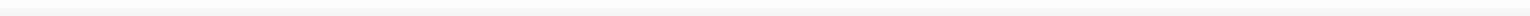• 展开全部Dijkstra(迪杰斯特拉)算法是典型的最短路径路由算法，用于计算一个节点到其他所有节e68a8462616964757a686964616f31333361316131最短路径。主要特点是以起始为中心向外层层扩展，直到扩展到终点为止。...
展开全部Dijkstra(迪杰斯特拉)算法是典型的最短路径路由算法，用于计算一个节点到其他所有节e68a8462616964757a686964616f31333361316131点的最短路径。主要特点是以起始点为中心向外层层扩展，直到扩展到终点为止。Dijkstra一般的表述通常有两种方式，一种用永久和临时标号方式，一种是用OPEN, CLOSE表方式用OPEN,CLOSE表的方式，其采用的是贪心法的算法策略，大概过程如下：1.声明两个集合，open和close，open用于存储未遍历的节点，close用来存储已遍历的节点2.初始阶段，将初始节点放入close，其他所有节点放入open3.以初始节点为中心向外一层层遍历，获取离指定节点最近的子节点放入close并从新计算路径，直至close包含所有子节点代码实例如下：Node对象用于封装节点信息，包括名字和子节点[java] view plain copypublic class Node {private String name;private Map child=new HashMap();public Node(String name){this.name=name;}public String getName() {return name;}public void setName(String name) {this.name = name;}public Map getChild() {return child;}public void setChild(Map child) {this.child = child;}}MapBuilder用于初始化数据源，返回图的起始节点[java] view plain copypublic class MapBuilder {public Node build(Set open, Set close){Node nodeA=new Node("A");Node nodeB=new Node("B");Node nodeC=new Node("C");Node nodeD=new Node("D");Node nodeE=new Node("E");Node nodeF=new Node("F");Node nodeG=new Node("G");Node nodeH=new Node("H");nodeA.getChild().put(nodeB, 1);nodeA.getChild().put(nodeC, 1);nodeA.getChild().put(nodeD, 4);nodeA.getChild().put(nodeG, 5);nodeA.getChild().put(nodeF, 2);nodeB.getChild().put(nodeA, 1);nodeB.getChild().put(nodeF, 2);nodeB.getChild().put(nodeH, 4);nodeC.getChild().put(nodeA, 1);nodeC.getChild().put(nodeG, 3);nodeD.getChild().put(nodeA, 4);nodeD.getChild().put(nodeE, 1);nodeE.getChild().put(nodeD, 1);nodeE.getChild().put(nodeF, 1);nodeF.getChild().put(nodeE, 1);nodeF.getChild().put(nodeB, 2);nodeF.getChild().put(nodeA, 2);nodeG.getChild().put(nodeC, 3);nodeG.getChild().put(nodeA, 5);nodeG.getChild().put(nodeH, 1);nodeH.getChild().put(nodeB, 4);nodeH.getChild().put(nodeG, 1);open.add(nodeB);open.add(nodeC);open.add(nodeD);open.add(nodeE);open.add(nodeF);open.add(nodeG);open.add(nodeH);close.add(nodeA);return nodeA;}}图的结构如下图所示：Dijkstra对象用于计算起始节点到所有其他节点的最短路径[java] view plain copypublic class Dijkstra {Set open=new HashSet();Set close=new HashSet();Map path=new HashMap();//封装路径距离Map pathInfo=new HashMap();//封装路径信息public Node init(){//初始路径,因没有A->E这条路径,所以path(E)设置为Integer.MAX_VALUEpath.put("B", 1);pathInfo.put("B", "A->B");path.put("C", 1);pathInfo.put("C", "A->C");path.put("D", 4);pathInfo.put("D", "A->D");path.put("E", Integer.MAX_VALUE);pathInfo.put("E", "A");path.put("F", 2);pathInfo.put("F", "A->F");path.put("G", 5);pathInfo.put("G", "A->G");path.put("H", Integer.MAX_VALUE);pathInfo.put("H", "A");//将初始节点放入close,其他节点放入openNode start=new MapBuilder().build(open,close);return start;}public void computePath(Node start){Node nearest=getShortestPath(start);//取距离start节点最近的子节点,放入closeif(nearest==null){return;}close.add(nearest);open.remove(nearest);Map childs=nearest.getChild();for(Node child:childs.keySet()){if(open.contains(child)){//如果子节点在open中Integer newCompute=path.get(nearest.getName())+childs.get(child);if(path.get(child.getName())>newCompute){//之前设置的距离大于新计算出来的距离path.put(child.getName(), newCompute);pathInfo.put(child.getName(), pathInfo.get(nearest.getName())+"->"+child.getName());}}}computePath(start);//重复执行自己,确保所有子节点被遍历computePath(nearest);//向外一层层递归,直至所有顶点被遍历}public void printPathInfo(){Set> pathInfos=pathInfo.entrySet();for(Map.Entry pathInfo:pathInfos){System.out.println(pathInfo.getKey()+":"+pathInfo.getValue());}}/*** 获取与node最近的子节点*/private Node getShortestPath(Node node){Node res=null;int minDis=Integer.MAX_VALUE;Map childs=node.getChild();for(Node child:childs.keySet()){if(open.contains(child)){int distance=childs.get(child);if(distanceminDis=distance;res=child;}}}return res;}}Main用于测试Dijkstra对象[java] view plain copypublic class Main {public static void main(String[] args) {Dijkstra test=new Dijkstra();Node start=test.init();test.computePath(start);test.printPathInfo();}}本回答由提问者推荐已赞过已踩过<你对这个回答的评价是？评论收起
展开全文• 这是使用BFS从(0,0)到(0，m-1)的矩阵中的最短路径的python实现 . 您可以将其更改为适合变量 .n,m,k1,k2=[int(i) for i in input().split()]arr=[[int(j) for j in input().split()] for i in range(n)]x=[[-1 for ...
这是使用BFS从(0,0)到(0，m-1)的矩阵中的最短路径的python实现 . 您可以将其更改为适合变量点 .n,m,k1,k2=[int(i) for i in input().split()]arr=[[int(j) for j in input().split()] for i in range(n)]x=[[-1 for i in range(m)] for j in range(n)]x=0vis={}q=[(0,0)]while len(q)!=0:curr=qrem=q.pop(0)vis[curr]=Truer=currc=currif r-1>=0 and arr[r-1][c]==0:if vis.get((r-1,c),-1)==-1 or vis[(r-1,c)]!=True:q.append((r-1,c))x[r-1][c]=x[r][c]+1if r+1if vis.get((r+1,c),-1)==-1 or vis[(r+1,c)]!=True:q.append((r+1,c))x[r+1][c]=x[r][c]+1if c-1>=0 and arr[r][c-1]==0:if vis.get((r,c-1),-1)==-1 or vis[(r,c-1)]!=True:q.append((r,c-1))x[r][c-1]=x[r][c]+1if c+1if vis.get((r,c+1),-1)==-1 or vis[(r,c+1)]!=True:q.append((r,c+1))x[r][c+1]=x[r][c]+1#for i in x:#print(i)ans=x[m-1]if ans==-1:print(-1)else:print(ans)输入矩阵应包含0 's and 1' s .  0表示可能的移动 .n是行数 .m是列数 .arr是给定的矩阵 .x是距离(0,0)的距离矩阵 .vis是一个字典，如果访问该节点则给出一个布尔值 .输出-1表示没有这样的路径可能 .
展开全文• 由于这是一个课程项目，时间比较急，而且自己不熟悉A*算法，所以参考网上的Dijkstra算法(http://blog.csdn.net/javaman_chen/article/details/8254309)的代码来实现了地图上任意两点最短路径的查询。但该demo存在...
地图上实现最短路径的查询，据我了解的，一般用Dijkstra算法和A*算法来实现。由于这是一个课程项目，时间比较急，而且自己不熟悉A*算法，所以参考网上的Dijkstra算法(http://blog.csdn.net/javaman_chen/article/details/8254309)的代码来实现了地图上任意两点的最短路径的查询。但该demo存在一个很严重的错误，缺了两行非常关键的代码……首先，来了解下Dijkstra算法：无向图的最短路径求解算法之——Dijkstra算法http://sbp810050504.blog.51cto.com/2799422/690803 。由此可以看出，Dijkstra算法的效率是很低的，它遍历的点很多，要以起始点为中心向外层层扩展，直到扩展到终点为止，所以数据量很少时不适合Dijkstra算法。处理该算法时，要特别注意在由一个点找到相邻该点最近点的时候，记得要将相邻的点的距离更新。Dijkstra一般的表述通常有两种方式，一种用永久和临时标号方式，一种是用OPEN, CLOSE表的方式，这里是采用第二种方式，也就是采用的贪心法的算法策略，大概过程如下：1.定义两个集合:open和close，open用于存储未遍历的节点，close用来存储已遍历的节点;2.初始阶段，将初始节点放入close，其他所有节点放入open;3.以初始节点为中心向外一层层遍历，获取离指定节点最近的子节点放入close并重新更新相邻点的距离，直至close包含所有子节点；此方法由一个点遍历了其他所有点，所以可以知道该点到其他所有点的距离，时间复杂度很高。那个demo是一个一个数据初始化的，我将它改为用数组存储，然后用for循环来初始化，也就是将它封装成我需要的数据接口，并没有很大的优化。算法核心只有两个函数：/*** 获取与node最近的子节点*/privateNode getShortestPath(Node node) {Node res= null;int minDis =Integer.MAX_VALUE;Map childs =node.getChild();//循环比较，找出离node最近的子节点for(Node child : childs.keySet()) {if(open.contains(child)) {int distance =childs.get(child);if (distance minDis=distance;res=child;}}}returnres;}View Codepublic voidcomputePath(Node start) {Node nearest= getShortestPath(start);//取距离start节点最近的子节点,放入closeif (nearest == null) {return;}close.add(nearest);open.remove(nearest);Map childs =nearest.getChild();for(Node child : childs.keySet()) {if (open.contains(child)) {//如果子节点在open中Integer newCompute = path.get(nearest.getName()) +childs.get(child);if (path.get(child.getName()) > newCompute) {//之前设置的距离大于新计算出来的距离path.put(child.getName(), newCompute);start.getChild().put(child, newCompute);close.add(start);pathInfo.put(child.getName(), pathInfo.get(nearest.getName())+ "-" +child.getName());}}}computePath(start);//重复执行自己,确保所有子节点被遍历computePath(nearest);//向外一层层递归,直至所有顶点被遍历}View Code那个demo漏了两行代码，就是找到最近的路径后，没有更新相邻点的距离start.getChild().put(child, newCompute);close.add(start);还有很多东西没优化，欢迎指出，一起学习！
展开全文• 本文主要讲述最短路径算法，一个主要原因是网上的“基于Matlab实现的两点之间最短路径算法”存在各种实现错误，目前为止还没有找到一个完全正确的。所以，本人改正相关错误，上传个正确的版本，即：采用Matlab实现的...
摘要
本文主要讲述最短路径算法，一个主要原因是网上的“基于Matlab实现的两点之间最短路径算法”存在各种实现错误，目前为止还没有找到一个完全正确的。所以，本人改正相关错误，上传个正确的版本，即：采用Matlab实现的两点之间Dijkstra(single path)算法。

1. Matlab源文件（dijkstra.m）

function [distance,path]=dijkstra(A,s,e)
%% 参数说明
%  邻接矩阵A
%  起点：s
%  终点：e
%  路径长： distance
%  路径：path

%% 算法主体
n=size(A,1);
D=A(s,:);
path=[];
visit=ones(1,n);
visit(s)=0;
parent=zeros(1,n);
for i=1:n-1
temp=zeros(1,n);
count=0;
for j=1:n
if visit(j)
temp=[temp(1:count) D(j)];
else
temp=[temp(1:count) inf];
end
count=count+1;
end
[value,index]=min(temp);
j=index; visit(j)=0;
for k=1:n
if D(k)>D(j)+A(j,k)
D(k)=D(j)+A(j,k);
parent(k)=j;
end
end
end

%% 结果输出（回溯法）
t = e;  %最短路径
while t~=s && t>0
path =[t,path];
p=parent(t);t=p;
end
path =[s,path];

if length(path)==1 % 最短路径长度
distance = A(s,e);
else
distance=D(e);
end

end

2. 测试

>> metrix=[
Inf     1   Inf   Inf   Inf   Inf   Inf   Inf   Inf   Inf   Inf     1
1   Inf     1   Inf   Inf   Inf   Inf   Inf   Inf   Inf   Inf     1
Inf     1   Inf     1   Inf   Inf   Inf   Inf   Inf     1   Inf   Inf
Inf   Inf     1   Inf     1   Inf   Inf     1   Inf   Inf   Inf   Inf
Inf   Inf   Inf     1   Inf     1   Inf   Inf   Inf   Inf   Inf   Inf
Inf   Inf   Inf   Inf     1   Inf     1   Inf   Inf   Inf   Inf   Inf
Inf   Inf   Inf   Inf   Inf     1   Inf     1   Inf   Inf   Inf   Inf
Inf   Inf   Inf     1   Inf   Inf     1   Inf     1     1   Inf   Inf
Inf   Inf   Inf   Inf   Inf   Inf   Inf     1   Inf   Inf   Inf   Inf
Inf   Inf     1   Inf   Inf   Inf   Inf     1   Inf   Inf     1   Inf
Inf   Inf   Inf   Inf   Inf   Inf   Inf   Inf   Inf     1   Inf     1
1     1   Inf   Inf   Inf   Inf   Inf   Inf   Inf   Inf     1   Inf]>> [distance, route]=dijkstra(metrix,1,1)
distance =
Inf
route =
1
>> [distance, route]=dijkstra(metrix,1,2)
distance =
1
route =
1     2
>> [distance, route]=dijkstra(metrix,1,7)
distance =
5
route =
1     2     3     4     8     7


展开全文• A*（A-Star)算法是一种静态路网中求解最短路最有 A star算法在静态路网中的应用 效的方法。 　公式表示为： f(n)=g(n)+h(n), 　其中 f(n) 是从初始经由节点n到目标的估价函数， 　g(n) 是在状态...
• Dijkstra算法实现两点之间最短路径算法[VC++
• Dijkstra(迪杰斯特拉)算法是典型的单源最短路径算法，用于计算一个节点到其他所有节点的最短路径。主要特点是以起始为中心向外层层扩展，直到扩展到终点为止。Dijkstra算法是很有代表性的最短路径算法，在很多专业...
• 其思路是将两点间距离分为过（指定的）第三点或是不过，然后取它们的最小值，如此循环就可以得到两点之间真正的最小值。 void floyd() { for (int k = 0; k < n; ++k) { for (int i = 0; i < n; ++i...
• 最短路径算法1.Dijkstra算法2.Bellman-Ford算法3.SPFA算法4.Floyd算法几种最短路径算法的对比Dijkstra算法、Bellman-Ford算法和SPFA算法的对比Dijkstra算法和Floyd算法的...多源最短路算法：求任意两点之间的最短路径.
• 两点之间最短路径的计算-floyd算法 Floyd算法的核心内容 算法过程图解 伪代码 完整代码 void floyd() { for (int k = 0; k < n; ++k) { for (int i = 0; i < n; ++i) { for (int j = 0; j < n; +...
• 两点之间只有一条路径，无需计算，当然用下述算法一样可以计算的出来，在蛋疼的情况下。二叉树图：再说多叉树，二叉树变种，顾名思义，每个点可以和N个点相连罢了。 同样，每两点之间只有一条路径，无需计算，...
• 使用动态规划计算任意两点间的最短路径，O（n^3）的复杂度，与基于Dijkstra的floyd算法复杂度一样， 但有些情况下有更好的性能 ...*功能:求任意两结点之间最短路径长度 */ #include "stdio.h" const int INFINITY =path
• 1 先求出原点离中间之间最短路径，然后，基于已经求出的最短路径，进一步求出它们之间最短路径。 转载于:https://www.cnblogs.com/wwwfj/p/3350166.html
• /* *求有向网中的任意两点的最短路径 *弗洛伊德算法的核心是 运用一个path二维数组 和一个A...*运用三层循环 （算法的核心） 计算任意两点之间最短路径长度 将经过的节点的下标存储在path数组中去 *递增的思想 *创...C语言数据结构
• 通过输入，能够实现找到最短路径。源代码能运行，简单易懂
• 两点之间只有一条路径，无需计算，当然用下述算法一样可以计算的出来，在蛋疼的情况下。 二叉树图： 再说多叉树，二叉树变种，顾名思义，每个点可以和N个点相连罢了。 同样，每两点之间只有一条路径，无需...
• MAX——定义两点之间若无路径赋予的最大值 变量： DIST[N]——存储已经搜寻到的最短路径 Is[N]——存储节点是否被遍历的状态 Path[N]——图之间的路径矩阵 Road[N]——存储最短路径时该节点的上一节点 算法...
• A星算法，计算任意座城市之间的最小路径。城市之间的路径为加权值，附上路径矩阵可随意更改路径值。从输出结果中可详细看到走过的城市和到该城市的最短路径值。
• Dijkstra算法是求源点到其它顶点的最短路径。怎样求任意个顶点之间最短路径
• Floyd-Warshall算法十字交叉法package com.geo.xiaojinku.udf.utils; import java.util.LinkedHashMap; import java.util.Map; import java.util.Map.Entry; im
• ## 最短路径算法

千次阅读 2020-07-01 00:18:45
图中每对点之间最短路径 Dijkstra 基本思想 ​ 狄克斯特拉算法解决图中一点到其余各点到最短路径的问题。其基本思想位：图G=(V,E)是一个有权有向图，把顶点V分成两组，第一组为已求出最短路径的点的集合(用S表示...
• Floyd算法Floyd是一种经典的多源最短路径算法，它通过动态规划的思想来寻找给定加权图中的多源点之间的最短路径，算法时间复杂度是O(n3)。之所以叫Floyd是因为该算法发明人之一是Robert Floyd，他是1978年图灵奖获得...
• 它是一种利用动态规划的思想寻找给定的加权图中多源点之间最短路径算法，采用带权邻接矩阵表示的图，通过一个三重循环，产生一个存储每个结点最短距离的矩阵。 众所周知，个顶点的最短距离会出现以下3种情况： ...数据结构 C语言
• 最短路径问题是图论研究中的一个经典算法问题， 旨在寻找图（由结点和路径组成的）中结点之间最短路径算法具体的形式包括： 确定起点的最短路径问题 - 即已知起始结点，求起始到所有最短路径的问题。 ...dijkstra floyd
• floyd最短路径算法是用于求图中任意两点之间最短路径的经典算法，但是绝大多数的讲解只是用三个循环简单带过。今天在学习spfa算法时有感得出其 可能 的内在逻辑。 图内有A,B点，以及N个点（包含A,B）。A到B的边数......